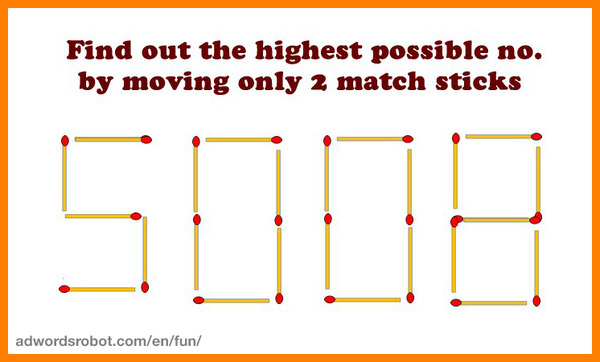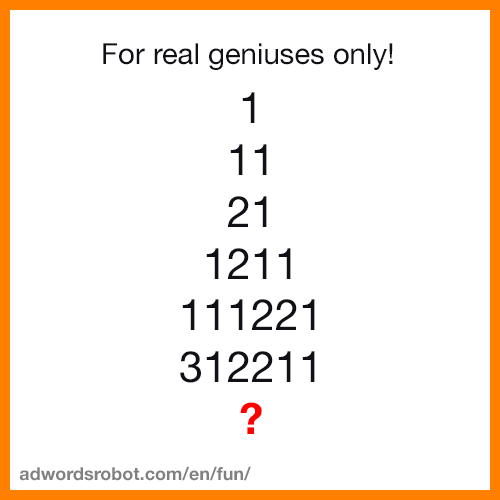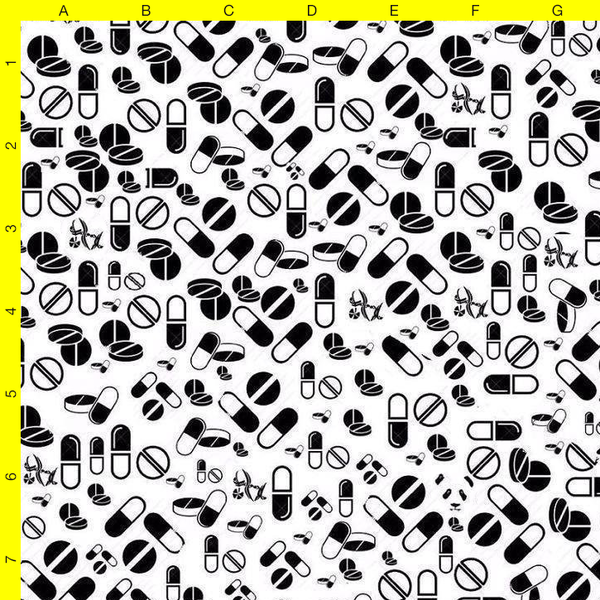## What is the largest number you can get by moving 2 matches?What is the largest number you can get by moving 2 matches?

``````Answer 1: 5 1 1 1 0 8
Answer 2: 8 0 1 1 1 5  (same as above, but rotated 180 degrees
Answer 3: 5 E 8 8      (5 * 10 ^ 88 = 5 with 88 zero's)
Answer 4: 5 /\ 1108    (/\ = ^ = power, a huge number with 775
digits)
``````

## What is the next number in sequence?What is the next number in sequence?

``````13112221

Just read how many of each digit you see in sequence on each line:
On the line above ? you see:

One Three, One One, Two Two(s), Two One(s).
``````

## Where is the panda?Where is the panda?

``````F6
``````